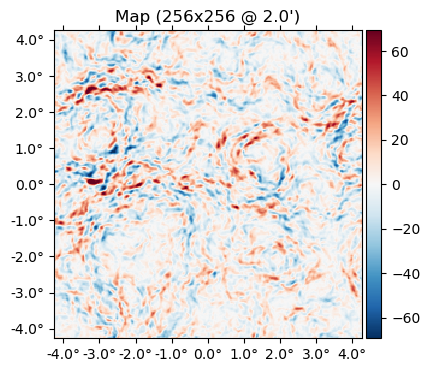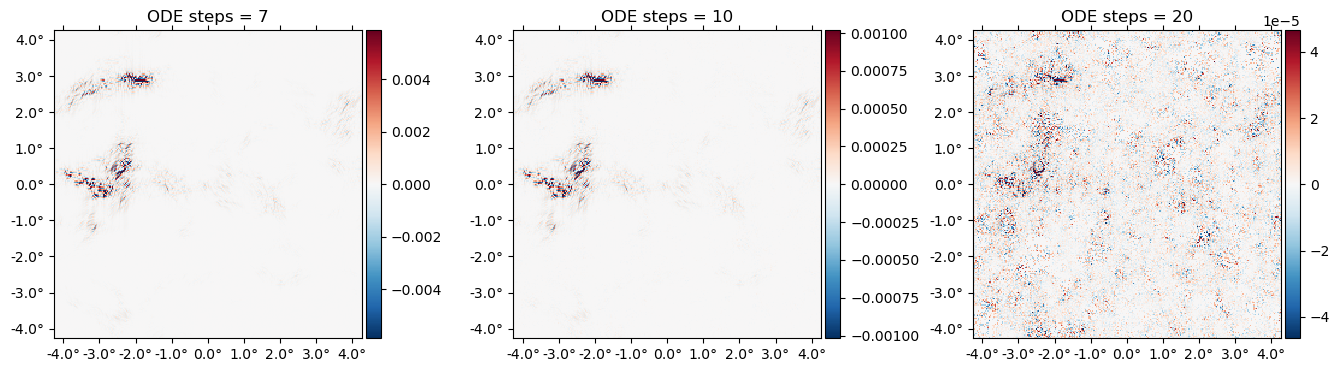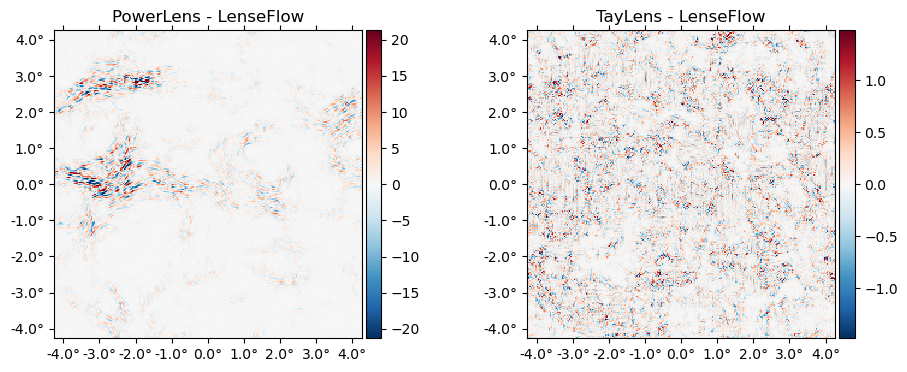# Lensing a flat-sky map

using CMBLensing, PyPlot

First we load a simulated unlensed field, $f$, and lensing potential, $\phi$,

@unpack f,ϕ = load_sim(
θpix  = 2,       # size of the pixels in arcmin
Nside = 256,     # number of pixels per side in the map
T     = Float32, # Float32 or Float64 (former is ~twice as fast)
pol   = :I       # :I for Intensity, :P for polarization, or :IP for both
);

We can lense the map with LenseFlow,

f̃ = LenseFlow(ϕ) * f;

And flip between lensed and unlensed maps,

animate([f,f̃], fps=1)

The difference between lensed and unlensed,

plot(f-f̃);CMBLensing flat-sky Field objects like f or ϕ are just thin wrappers around arrays. You can get the underlying data arrays for $I(\mathbf{x})$, $Q(\mathbf{x})$, and $U(\mathbf{x})$ with f[:Ix], f[:Qx], and f[:Ux] respectively, or the Fourier coefficients, $I(\mathbf{l})$, $Q(\mathbf{l})$, and $U(\mathbf{l})$ with f[:Il], f[:Ql], and f[:Ul],

mapdata = f[:Ix]
256×256 view(::Array{Float32, 3}, :, :, 1) with eltype Float32:
-52.4875    -44.1927    -20.8745   …   -50.7907    -47.514     -48.5475
-41.8072    -34.6056    -12.1086       -46.549     -38.5433    -36.6914
-32.488     -22.8599     -6.95026      -35.8732    -33.1676    -32.2153
-23.5905    -15.81      -12.1051       -22.8456    -26.7244    -27.0778
-9.26323    -6.65683   -18.7204       -14.9415    -17.5103    -14.9762
5.47059     5.88917    -8.53401  …    -8.36921    -2.75452     1.52032
23.509      24.434      17.0082         5.18877    22.9085     25.879
57.5089     58.3714     54.9225        23.8288     54.0946     60.8511
94.6499     99.2414     97.2802        43.2281     77.5148     90.2145
112.88      126.822     128.739         60.1666     86.5743     99.3174
113.398     131.968     135.8      …    76.9052     91.131      97.6228
104.947     118.78      115.517         90.0779     96.4933     94.3344
89.933      94.4758     85.7985        97.8001     98.591      88.5233
⋮                                ⋱                             ⋮
-178.666    -123.581     -78.9616      -253.029    -242.646    -221.115
-187.731    -137.613    -101.847    …  -245.117    -241.921    -226.815
-200.956    -156.606    -125.526       -246.547    -247.496    -235.856
-206.041    -164.962    -137.589       -242.803    -250.456    -240.135
-186.452    -158.567    -145.481       -218.049    -231.628    -218.448
-156.184    -146.205    -151.194       -174.619    -187.523    -177.052
-134.914    -134.874    -145.602    …  -134.557    -143.428    -141.145
-126.211    -125.708    -130.125       -107.085    -115.054    -122.454
-114.548    -106.611     -97.6413       -84.8219    -97.2973   -110.23
-94.8088    -75.6119    -54.6598       -69.2433    -83.5316    -95.1499
-74.9895    -52.3557    -27.7943       -59.9018    -71.7007    -80.7054
-62.3109    -46.2644    -23.2328   …   -53.3054    -59.3069    -64.5931

If you have your own map data in an array you'd like to load into a CMBLensing Field object, you can construct it as follows:

FlatMap(mapdata, θpix=3)
65536-element 256×256-pixel 3.0′-resolution LambertMap{SubArray{Float32, 2, Array{Float32, 3}, Tuple{Base.Slice{Base.OneTo{Int64}}, Base.Slice{Base.OneTo{Int64}}, Int64}, true}}:
-52.487473
-41.80719
-32.488003
-23.590511
-9.263233
5.4705887
23.508987
57.50889
94.64986
112.880066
113.397896
104.94669
89.932976
⋮
-221.11548
-226.81479
-235.85616
-240.13464
-218.44809
-177.0516
-141.14462
-122.45426
-110.23017
-95.1499
-80.70537
-64.59307

For more info on Field objects, see Field Basics.

## Inverse lensing

You can inverse lense a map with the \ operator (which does A \ b ≡ inv(A) * b):

LenseFlow(ϕ) \ f;

Note that this is true inverse lensing, rather than lensing by the negative deflection (which is often called "anti-lensing"). This means that lensing then inverse lensing a map should get us back the original map. Lets check that this is the case:

Ns = [7 10 20]
plot([f - (LenseFlow(ϕ,N) \ (LenseFlow(ϕ,N) * f)) for N in Ns],
title=["ODE steps = $N" for N in Ns]);A cool feature of LenseFlow is that inverse lensing is trivially done by running the LenseFlow ODE in reverse. Note that as we crank up the number of ODE steps above, we recover the original map to higher and higher precision. ## Other lensing algorithms We can also lense via: • PowerLens: the standard Taylor series expansion to any order: $$$f(x+\nabla x) \approx f(x) + (\nabla f)(\nabla \phi) + \frac{1}{2} (\nabla \nabla f) (\nabla \phi)^2 + ...$$$ • TayLens (Næss&Louis 2013): like PowerLens, but first a nearest-pixel permute step, then a Taylor expansion around the now-smaller residual displacement plot([(PowerLens(ϕ,2)*f - f̃) (Taylens(ϕ,2)*f - f̃)], title=["PowerLens - LenseFlow" "TayLens - LenseFlow"]);## Benchmarking LenseFlow is highly optimized code since it appears on the inner-most loop of our analysis algorithms. To benchmark LenseFlow, note that there is first a precomputation step, which caches some data in preparation for applying it to a field of a given type. This was done automatically when evaluating LenseFlow(ϕ) * f but we can benchmark it separately since in many cases this only needs to be done once for a given$\phi$, e.g. when Wiener filtering at fixed$\phi\$,

using BenchmarkTools
@benchmark cache(LenseFlow(ϕ),f)
BenchmarkTools.Trial: 110 samples with 1 evaluation.
Range (min … max):  43.978 ms … 49.619 ms  ┊ GC (min … max):  8.97% … 16.42%
Time  (median):     44.988 ms              ┊ GC (median):     9.01%
Time  (mean ± σ):   45.597 ms ±  1.639 ms  ┊ GC (mean ± σ):  10.58% ±  2.95%

▁▁ ▄▁▁ ▁▁▇▄█
██▅███▅██████▆▆▆▃▅▁▁▅▅▁▁▁▁▁▁▁▃▁▁▁▁▁▁▁▁▁▁▁▁▁▁▃▅▅▃▆▁█▃▅▃▅▁▃▁▃ ▃
44 ms           Histogram: frequency by time        49.5 ms <

Memory estimate: 92.42 MiB, allocs estimate: 1743.

Once cached, it's faster and less memory intensive to repeatedly apply the operator:

@benchmark Lϕ * f setup=(Lϕ=cache(LenseFlow(ϕ),f))
BenchmarkTools.Trial: 63 samples with 1 evaluation.
Range (min … max):  31.697 ms … 38.788 ms  ┊ GC (min … max): 0.00% … 10.77%
Time  (median):     32.929 ms              ┊ GC (median):    0.00%
Time  (mean ± σ):   33.514 ms ±  1.763 ms  ┊ GC (mean ± σ):  2.51% ±  4.53%

▅ ▅▂▂▅▂▂   ▅█                                        ▂
▅▁████████▅█▁████▁█▅▁▁█▁▁▅▁▁▁▅▁▁▁▁▁▁▁▁▁▁▁▁▁▁▁▁▅▅▅▁▅▅▁▅▁█▅▁█ ▁
31.7 ms         Histogram: frequency by time        37.1 ms <

Memory estimate: 16.13 MiB, allocs estimate: 441.

Note that this documentation is generated on limited-performance cloud servers. Actual benchmarks are likely much faster locally or on a cluster, and yet (much) faster on GPU.